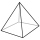# Pythagorean theorem + volume - math problems

#### Number of problems found: 155

• Right pyramidA right pyramid on a base 4 cm square has a slanted edge of 6 cm. Calculate the volume of the pyramid.
• Tetrahedral pyramidCalculate the surface S and the volume V of a regular tetrahedral pyramid with the base side a = 5 m and a body height of 14 m.
• Cube diagonalsCalculate the length of the side and the diagonals of the cube with a volume of 27 cm3.
• Two ballsTwo balls, one 8cm in radius and the other 6cm in radius, are placed in a cylindrical plastic container 10cm in radius. Find the volume of water necessary to cover them.
• Slant heightThe cone's slant height is 5cm, and the radius of its base is 3cm, find the volume of the cone.
• 3s prismIt is given a regular perpendicular triangular prism with a height of 19.0 cm and a base edge of 7.1 cm. Calculate the volume of the prism.
• Triangular pyramidWhat is the volume of a regular triangular pyramid with a side 3 cm long?
• Pyramid heightFind the volume of a regular triangular pyramid with edge length a = 12cm and pyramid height h = 20cm.
• The volumeThe volume of the cone is 94.2 dm³, the radius of the base is 6 dm. Calculate the surface of the cone.
• ConeCalculate the volume of the rotating cone with a base radius 26.3 cm and a side 38.4 cm long.
• The conicalThe conical candle has a base diameter of 20 cm and a side of 30 cm. How much dm ^ 3 of wax was needed to make it?
• TetrahedronCalculate height and volume of a regular tetrahedron whose edge has a length 6 cm.
• Hexagon cut pyramidCalculate the volume of a regular 6-sided cut pyramid if the bottom edge is 30 cm, the top edge is 12 cm, and the side edge length is 41 cm.
• Secret treasureScouts have a tent in the shape of a regular quadrilateral pyramid with a side of the base 4 m and a height of 3 m. Find the container's radius r (and height h) so that they can hide the largest possible treasure.
• Solid cuboidA solid cuboid has a volume of 40 cm3. The cuboid has a total surface area of 100 cm squared. One edge of the cuboid has a length of 2 cm. Find the length of a diagonal of the cuboid. Give your answer correct to 3 sig. Fig.

Do you have an exciting math question or word problem that you can't solve? Ask a question or post a math problem, and we can try to solve it.

We will send a solution to your e-mail address. Solved examples are also published here. Please enter the e-mail correctly and check whether you don't have a full mailbox.

Tip: Our volume units converter will help you with the conversion of volume units. Pythagorean theorem is the base for the right triangle calculator. Pythagorean theorem - math problems. Volume - math problems.